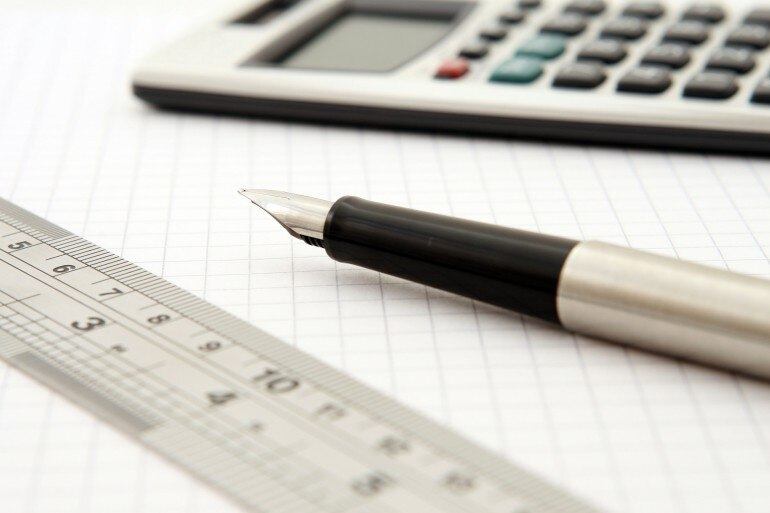## Sidebar

### 4 steps to solve even the toughest math problem and improve your math skills

#### Mathematics is a field where one can take multiple approaches to get to the solution of a problem. This simplified step-by-step approach will help you unravel the solution to even the toughest math problem.

There are multiple ways to solve math problems; however, a simplified method that can help everyone to solve even the toughest problem is a three-step process.

The process is:

1. Visualize the problem
2. Approach to be followed for that problem
3. Lastly, solve the problem

Here are four steps to help solve any math problems easily:

### 1. Read carefully, understand, and identify the type of problemDefine the category in which your math problem fits in before you move forward as it will help find the best solution to solve it.

When you first start studying math, check the type of problem -- whether it is a word problem, of concerning fractions, quadratic equations, or any other type.

Define the category in which your math problem fits in before you move forward as it will help with the best solution to solve it.

Reading the problem carefully and ensuring that you have understood the problem correctly is extremely important for taking the next steps.

### 2. Draw and review your problemYou could also probably look for patterns or make use of graphs to solve the math problem.

Once you have understood the problem, the next step could be to draw the problem as it will help you with the way forward. The drawing can be simple in form of shapes or shapes with numbers.

Here you could also probably look for patterns or make use of graphs. Once this entire process of understanding, reading and drawing is conducted you need to review the analysis you have made out of it.

This will help you to decide the type of problem and the method to solve it.

### 3. Develop the plan to solve itFirstly one needs to figure out the formula you will need to solve the math problem.

There are four simple steps which one needs to go through in order to develop a plan to solve it. The steps are as mentioned below:

• Firstly one needs to figure out the formula you will need to solve the problem. Here you need to spend some time reviewing the concepts in your textbooks which will help you solve the problem
• You need to write down your need in order to get the answer to your problem. For this, you need to make a step-by-step list of the things which you need to solve the problem and also help you to stay organised
• In case there is an easier problem which is available then you could probably work on that first to solve it. Sometimes, the formulas are repetitive for solving both the problems. This will give you some more time to solve the difficult problem
• You can make an educated guess about the answer so that you can try and get the estimate the answer before you start to solve it. Here you can identify the number and other factors as well that will contribute to the same. Lastly, review the estimate and then check if you haven't left out on anything

### 4. Solve the problemEnsure that all the steps which you had listed to solve the math problem are completed

Once your strategy and method to solve the problem are ready you could start solving it. The steps are as below:

• Ensure that all the steps which you had listed to solve the problem are completed. Cross check each of your answers to ensure that the accuracy is perfect
• Compare the answer with the estimates which you have listed after completion of each and every step. This will have you to save time in case the end result is not what you were looking for. Also check if you have completed all the steps carefully
• In case you realize in the middle that your plan isn't working then you can always go back to the planning stage and make a new plan. Sometimes due to common mistakes, this happens but you should learn to accept it and be ready with Plan B to solve it
• Once you have solved the problem correctly, you should go back and look at the process. Take a moment to reflect on the problem and the method through which you have solved it. This will help in identifying concepts that you need to learn while practicing.

- Article by Sudhanshu Sinhal, Managing Director, Sinhal Classes Pvt. Ltd.